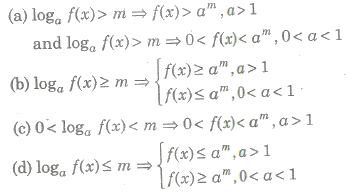# Logarithm Notes Class 11th Maths

0
1896

If a is a positive real number other than 1 and ax = m, then x is called the logarithm of m to the base a, written as loga m. In loga m, m should be always positive.

(i) If m < 0, then loga m will be imaginary and if m = 0, then loga m will be meaningless.

(ii) loga m exists only, if m, a > 0 and a ≠ 1.

Types of Logarithms

1. Natural or Napier Logarithms

The logarithms with base ‘e’ (e = 2.718) are called natural logarithms.

e.g., loge x, loge 25 etc.

2. Common or Brigg’s Logarithms

The logarithm with base ’10’ are called common logarithm.

e.g., log10 x, log10 75 etc.

Note In a logarithmic expression when the base is not mentioned, it is taken as 10.

Characteristic and Mantissa of a Logarithm

The logarithm of positive real number ‘n’ consists of two parts.

1. The integral part is known as the characteristic. It is always an integer positive, negative or zero.
2. The decimal part is called as the mantissa. The mantissa is never negative and is always less than one.

< .strong>To Find the Characteristic

Case I. When the number is greater than 1.

The characteristic is one less than the number of digits in the left of decimal point in the given number.

e.g., 6.125 characteristic is O.

61.321 characteristic is 1.

725.132 characteristic is 2.

Case II. When the number is less than 1.

The characteristic is one more than the number of zero, between the decimal point and the fu-st significant digits of the number and it is negative.

e.g.. 0.7. characteristic is 1.

0.06712 characteristic ia 2.

0.00031 characteristic is 4.

Note In place of -1 or -2 etc.. we use 1 tone bar) and 2 (two bar) etc.

Properties of Logarithm

I. Characteristic of the logarithm of any number greater than I will be less than number of digits in the ‘integral part of the number’.

2. Characteristic of the logarithm of a number less than one negative and is one more than the number of zeros to the right of the decimal point in the number.

3. Since. a negative number can never be expressed as the power of 10, mantissa should always be kept positive. Hence, whenever characteristic ig negative, minus sign is placed above the characteristic and not to i. to show that the mantissa is always positive.

4. Mantissa of the logarithm of the number having same digits in the same order will be the same, irrespective of the position of the decimal point.

Anti-Logarithm

The positive number a is called the anti-logarithm of a number 6, if a Is anti-logarithm of b. then we write a = antilog b.

So, a = antilog b ⇔ log a = b

Important Results on Logarithm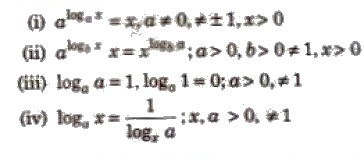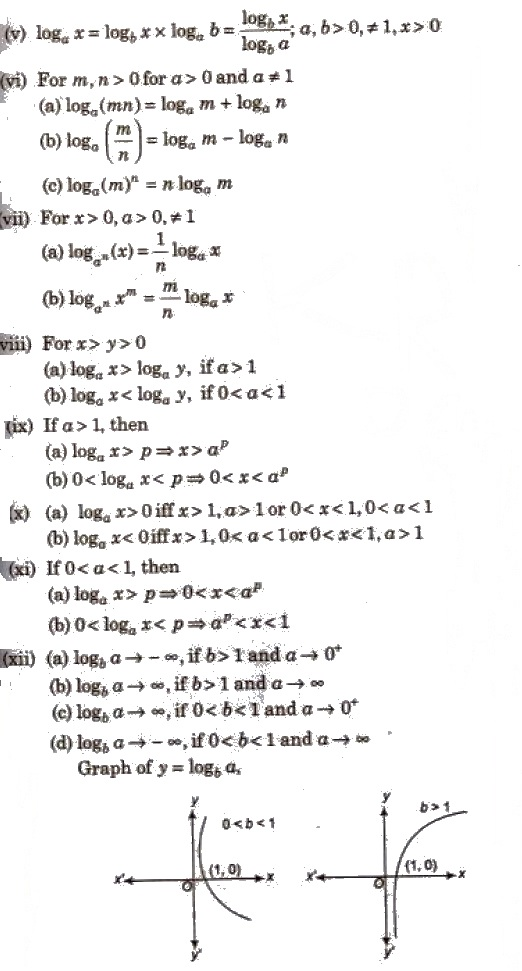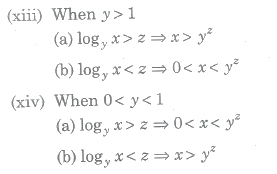Logarithmic Inequalities

(i) Let a is a real number such that a > 0, ≠ 1, then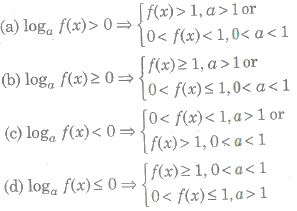(ii) Let a is a real number, then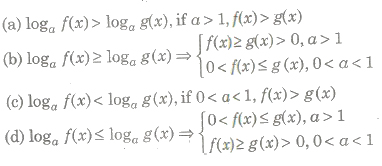(iii) Let a is a real number such that a > 0, ≠ 1, then (a)